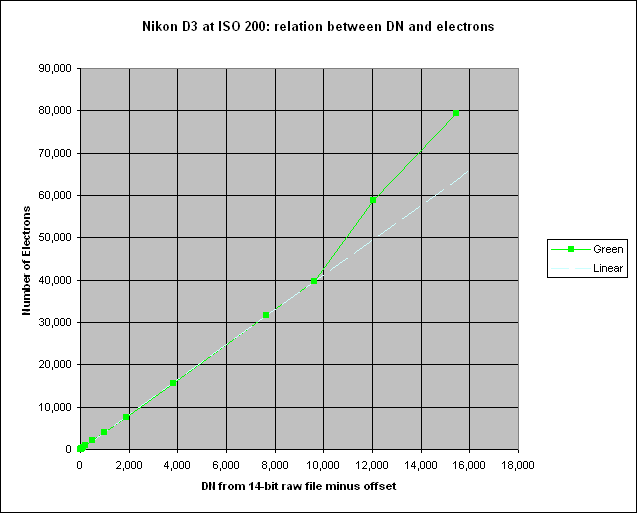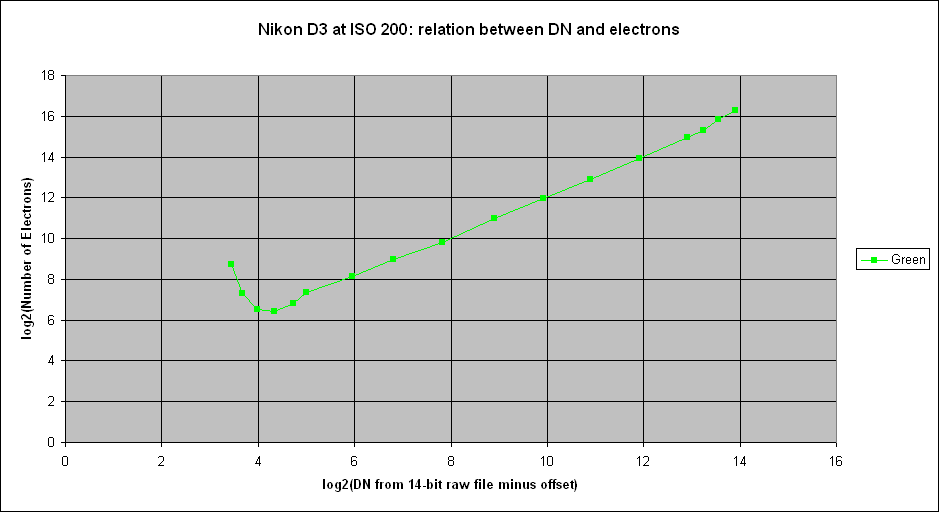Up to Nikon D3 index

# Nikon D3 gain and full well capacity at ISO 200

See here.

## Results

The table below shows statistics of a 200 x 200 pixel area in the centre of each image for the green component after the two frames have been subtracted.

It should be noted that the top five exposures are at or close to saturation, and this is the reason why their standard deviation is lower.

The number of electrons is calculated by squaring the signal-to-noise ratio, and the latter is calculated after quadratically subtracting the read noise from the measured noise.

The bottom two exposures are into the region where the D3 clips the noise at zero. This results in an underestimate of the noise and hence, when the read noise is subtracted off, to negative values for the number of electrons.

The gain is calculated by dividing the number of electrons by the Mean DN. It is plotted below:Ideally this should be a straight, horizontal line because the gain is, ex hypothesi, independent of exposure. It should vary only as a function of ISO setting.

The gain goes wild at low exposures, and would be wilder still had I not suppressed the points close to the origin. This is ok: it becomes increasingly difficult to measure the gain accurately as exposure reduces.

The jump in the gain at a shutter speed of 1/4 second is due to an anomaly in the Mean DN data, see nasty kink here.

I prefer to take, as a fair estimate of the gain, the middle portion of the graph. Thus the gain is about 4.2 electrons per DN.

A better estimate of the gain can be got from plotting the number of electrons against DN:The white line shows the result of a linear regression on the twelve points between DN=20 and DN=9633. This region excludes the inaccurate points at the low end and also the kink at the high end (which I don't trust because I have no explanation for it). The result, with intercept constrained to be zero, is:

Number of electrons = 4.1218 * DN, R² = 0.9999

Hence I measure the gain of the Nikon D3 as 4.1 electrons per DN, in the green component of the Bayer array, when written to 14-bit NEF files.

Since the mean DN at green saturation is 15907.6 (from table above), it follows that the full well capacity of the Nikon D3 is 15907.6 * 4.1218 = 65,568 electrons.

The full well capacity of the blue and red components cannot be measured due to firmware clipping.

In case anyone is thinking "well, with that kink in the curve, I don't trust this guy's measurement at all", I plot below the same data on logarithmic scales:This shows the kink (at the righthand end) in a better perspective.

The "hockey stick" effect at the left end is caused by the D3 clipping the noise at the low end, resulting in a lower noise estimate, and hence a higher estimate for the number of electrons.

Peter Facey, Winchester, England
20080123 originated